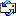﻿ Creator and Redeemer

God is both our Creator and Redeemer

The Creator is God Almighty, the Creator of the Universe.  Humanity is created by one Creator. Creation originate from the Creator.  God is creator to create that creator.  We came to the world through different wombs, but the source is same creator.  We cried when .  The whole creation shows the wonder of a Creator.  Creation is the work of eternal Creator.

God's accuracy may be observed in the hatching of eggs

• the eggs of the potato bug hatch in 7 days;
• those of the canary in 14 days;
• those of the barnyard hen in 21 days;
• The eggs of ducks and geese hatch in 28 days;
• those of the mallard in 35 days;
• The eggs of the parrot and  the ostrich hatch in 42 days.
(Notice, they are all divisible by seven, the number of days in a week.)

God's wisdom is seen in the making of an elephant.  The four legs of this great beast all bend forward in the same direction.  No other quadruped is so made. God planned that this animal would have a huge body, too large to live on two legs.  For this reason He gave it four fulcrums so that it can rise from the ground easily.

• The horse rises from the ground on its two front legs first.

• A cow rises from the ground with its two hind legs first. How wise the Lord is in all His works of creation!

God's wisdom is revealed in His arrangement of sections and segments, as well as in the number of grains.

• Each watermelon has an even number of stripes on the rind.

• Each orange has an even number of segments.

• Each ear of corn has an even number of rows.

• Each stalk of wheat has an even number of grains.

• Every bunch of bananas has on its lowest row an even number of bananas, and each row decreases by one, so that one row has an even number  and the next row an odd number.

• The waves of the sea roll in on shore twenty-six to the minute in all kinds of weather.

• All grains are found in even numbers on the stalks, and the Lord specified thirty fold, sixty fold, and a hundred fold all even numbers.

God has caused the flowers to blossom at certain specified times during the day. Linnaeus, great the botanist, once said that if he had a conservatory  containing the right kind of soil, moisture and temperature,  he could tell the time of day or night by the flowers that were open and those that were closed !

 9 X 1 = 09 9 X 2 = 18 9 X 3 = 27 9 X 4 = 36 9 X 5 = 45 9 X 6 = 54 9 X 7 = 63 9 X 8 = 72 9 X 9 = 81 9 X 10 = 90

1 x 1 = 1
11 x 11 = 121
111 x 111 = 12321
1111 x 1111 = 1234321
11111 x 11111 = 123454321
111111 x 111111 = 12345654321
1111111 x 1111111 = 1234567654321
11111111 x 11111111 = 123456787654321
111111111 x 111111111 = 12345678987654321

1 x 8 + 1 = 9
12 x 8 + 2 = 98
123 x 8 + 3 = 987
1234 x 8 + 4 = 9876
12345 x 8 + 5 = 98765
123456 x 8 + 6 = 987654
1234567 x 8 + 7 = 9876543
12345678 x 8 + 8 = 98765432
123456789 x 8 + 9 = 987654321

1 x 9 + 2 = 11
12 x 9 + 3 = 111
123 x 9 + 4 = 1111
1234 x 9 + 5 = 11111
12345 x 9 + 6 = 111111
123456 x 9 + 7 = 1111111
1234567 x 9 + 8 = 11111111
12345678 x 9 + 9 = 111111111
123456789 x 9 +10= 1111111111

9 x 9 + 7 = 88
98 x 9 + 6 = 888
987 x 9 + 5 = 8888
9876 x 9 + 4 = 88888
98765 x 9 + 3 = 888888
987654 x 9 + 2 = 8888888
9876543 x 9 + 1 = 88888888
98765432 x 9 + 0 = 888888888

142857 X 1 = 142857

142857 X 2 = 285714

142857 X 3 = 428571

142857 X 4 = 571428

142857 X 5 = 714285

142857 X 6 = 857142

142857 X 7 = 999999
142 + 857 = 999

14 + 28 + 57 = 99
142857 X 142857 = 20408122449
20408 + 122449 = 142857

<4> 142857×9＝1285713

<1> 142857×10＝1428570

<8> 142857×11＝1571427

<5> 142857×12＝1714284

<2> 142857×13＝1857141

（9 >>>）142857×14 ＝ 1999998

1＋4＋2＋8＋5＋7 ＝ 27
2＋7 ＝ 9
3 X 9 = 27
4+8+9+6+5 = 32
3+2 = 5
6+7+3+2 = 18， 1+8 = 9

The perfect orchestration of the symphony of life is one of the Creator's greatest and most beautiful miracles.  The Creator didn’t make love conditional, love is something that belongs to us alone and enables us to flee the Creator.  We are not sheep or cows, God didn’t create fences for us or boundaries to contain our nationalities.  We were all born to be peaceful citizens of the world.  A man of God would never burn or harm a temple of any kind - regardless of religion.